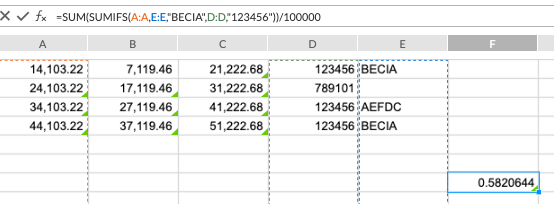## コメント

5件のコメント

•Hi Kellie!

Looking at that, it would seem that you are missing a second criteria range before the criteria "123456". Something like:

`=SUM(SUMIFS('Essbase'!\$E:\$E,'Essbase'!\$A:\$A,"BECIA",CRITERIA_RANGE_HERE,"123456"))/1000000`

I was able to get a calculation applied that worked. Here's an example:Let me know what follow-ups you have for me. Chat soon, and happy Friday!

•Mike, the formula I sent you was a bad example, I apologize.  What I am actually trying to do is sum multiple criteria, so if column D is 123456 or 789101, add the value in column C.  In Excel it would be the below formula, but in spreadsheets the below formula gives #VALUE.  I would expect the below formula to result in \$144,890.72.  It seems that in spreadsheets, it wants me to create individual sumif formulas for the 123456 and another for the 789101 and to add them together.  Are you able to get a result if you try this?  I am not able. Thank you Mike!

=SUM(SUMIF(\$C:\$C,{"123456","789101"},\$D:\$D))

•Correction on my above formula =SUM(SUMIF(\$D:\$D,{"123456","789101"},\$C:\$C)), sorry again! This is what I am trying to accomplish.

•Gotcha, Kellie. I see what you are trying to do there. I believe this fails in Workiva as Array formulas are not yet support (the portion of the SUMIF that is {"123456","789101"}).

A way around this would be to sum together multiple SUMIFS, such as...

`=SUMIF(D:D,"123456",C:C)+SUMIF(D:D,"789101",C:C)`

A SUMIFS would only work if the criteria were the same, like an AND function, but not for an OR type function. Does that make sense?

•Mike, yes that does make sense, thank you for the feedback!

サインインしてコメントを残してください。